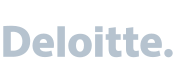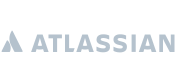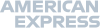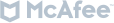# Introduction to R

• 1 Day
• Beginner
• Virtual | Classroom
• £ On Request

This is an intensive course for developers interested in R

Getting started with R

Importing data from Excel, SAS and CSV

Creating graphics with R

Summarising complex data sets using the tidyverse

### Overview

This is a one day intensive course on R. The course is a mixture of lectures and computer practicals. The main focus will be to introduce fundamental R concepts. No prior programming knowledge of any kind is assumed. This course is suitable for a wide range of applicants e.g., biologists, statisticians, engineers, students.

### Outline

• Introduction to R: A brief overview of the background and features of the R statistical programming system.
• Data entry: A description of how to import and export data from R.
• Data types: A summary of R's data types.
• R environment: A description of the R environment including the R working directory, creating/using scripts, saving data and results.
• R graphics: Creating, editing and storing graphics in R.
• Summary statistics: Measures of location and spread.
• Manipulating data in R: Describing how data can be manipulated in R using logical operators.
• Vector operations: details of R's vectors operations.